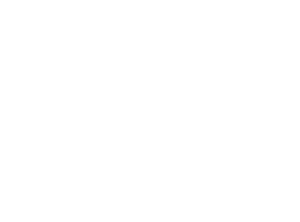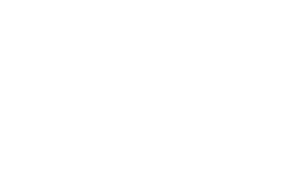# Year 1 Spring 2 Maths Challenge

Hi Year 1 your Spring 2 Maths challenges are as follows:

Red:What is 1 more than 9?

What is 1 more than 7?

What is 1 more than 5?

Yellow and Orange:

What is 1 less than 8?

What is 1 less than 12?

What is is 1 less than 16?

Blue and Green:

What is 1 more than 19?

What is 1 less than 12?

What comes next?A challenge for all of Year 1. Please use the vocabulary below to describe the position of the 3D shapes:

Next to    below     above    inside

1. The sphere is _____________ the pyramid.
2. The cone is ______________ the cylinder.
3. The cube is ______________ the cone.
4. The black dotted lines are ________ the pyramid and cone.

1. #### Mohammed Umar Hussain

1.one more than 19 is 20.
2.one less than 12 is 11.
3.one more than 12 is 13.
1.the sphere is above the pyramid.
2.the cone is above the cylinder.
3.the cube is next to the cone.
4.the black dotted lines are inside the pyramid and cone

2. #### Marwa Haji

Green group
1. what is 1 less then 20? 19
The sphere below the pyramid.
The cone above the cilender.

3. #### Rahima Sultan Dufa

What is 1 more than 19? More than 19 is 20
What is 1 less than 12? less than 12 is 11.
Next to below above inside
The sphere is below the pyramid.
The cone is above the cylinder.
The cube is next to the cone.
The black dotted lines are inside the pyramid and cone.

Blue and green
What is 1 more than 19? 20
What is 1 less than 12? 11
yellow and orange
What is 1 less than 8? 7
What is 1 less than 12? 10
What is 1 less than 16? 15
Red
What is 1 more than 9? 10
What is 1 more than 7? 8
What is 1 more than 5? 6

5. #### Sabah Batool Shah

Red
What is 1 more than 9? 10
What is 1 more than 7? 8
What is 1 more than 5? 6

Yellow and Orange:
What is 1 less than 8? 7
What is 1 less than 12? 11
What is is 1 less than 16? 15

Blue and Green:
What is 1 more than 19? 20
What is 1 less than 12? 11

Challenge :
1.The sphere is below the pyramid.
2.The cone is above the cylinder.
3.The cube is next to the cone.
4.The black dotted lines are inside the pyramid and cone.

6. #### Merab Muheen Rehman

1.What is 1 more than 19?20
2.What is 1 less than 12?11
3.What comes next?12
Challenge:
The sphere is ____below____the pyramid.
The cone is ______Next to____ the cylinder.
The cube is ______inside______ the cone.
The black dotted lines are _____above___ the pyramid and cone.

7. #### Imran Namdar

yellow and orange:
one less than 8 is 7
one less than 12 is 11
one less than 16 is 15
one more then 9 is 8

8. #### Seifu Roba

Yellow and Orange:
What is 1 less than 8?=7
What is 1 less than 12?=11
What is is 1 less than 16?=15

9. #### Asilah Kauser

Red:
10.
8.
6.

Yellow and orange:
7.
11.
15.

Blue and green:
20.
11.
13.

• #### Asilah Kauser

Challenge:
1. The sphere is below the pyramid.
2. The cone is above the cylinder.
3. The cube is next to the cone.
4. The black dotted lines are inside the pyramid and cone.